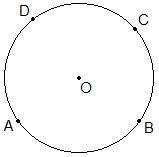# Circumference of a Circle | Perimeter of Circle## Circumference of a Circle Definition

The curved boundary or rim of a circle which is equidistant from its centre is called the circumference of a circle. The circumference of a circle is also known as the perimeter of circle.

**********************

10 Math Problems officially announces the release of Quick Math Solver, an android APP on the Google Play Store for students around the world.**********************In the given figure curve line ABCD is the circumference of the circle. It is generally denoted by the letter C.

### How to find circumference of a circle formula?

Let us do an experiment. Take a coin. Put it on the scale and roll it as shown in the figure below.When rolling completes one round it travels the length equal to the circumference of the coin. Repeat this activity for other circular objects.

In such activity, if the diameter of the coin is 3.5cm then the circumference will be 11cm. If the diameter is 7cm, the circumference will be 22cm. Similarly, if the diameter is 14cm, the circumference will be 44cm.

From this experiment, we can find that the ratio of circumference to diameter is always the same. i.e.

Circumference/Diameter = 11/3.5 = 22/7 = 44/14 = 3.14

This constant ratio of circumference to diameter (C/d) = 3.14 is denoted by the Greek symbol π (pi).

i.e.    C/d = π

or,     C = πd

or,     C = π × 2r  [ d = 2r]

or,     C = 2πr

Therefore, we have the following Circumference Formula,

Circumference of a circle using radius(r) of the circle,

C = 2πr

Circumference of a circle using diameter(d),

C = πd

If circumference is given, we can find the radius of a circle from the circumference by applying the first formula and we can find diameter from the circumference by applying the second formula.

### Perimeter of semicircle

The total outer length of a semicircle (half circle) is called the perimeter of semicircle. It is given by the sum of half of the circumference and diameter of the circle.From the figure of semicircle given above,

Perimeter   = C/2 + d

= 2πr/2 + 2r

= πr + 2r

= r(π + r)

Therefore, Perimeter of Semicircle Formula is given by,

Perimeter = r(π + r)

### Worked Out Examples

Example 1: Find the circumference of the circle having a radius 3.5cm.

Solution: Here,

Circumference (C) = ?

We know,

Circumference of circle,

= 2πr

= 2 × 22/7 × 3.5cm

= 22cm

The circumference of circle is 22cm. Ans.

Example 2: Find the circumference of the circle having a diameter 14cm.

Solution: Here,

Diameter (d) = 14 cm

Circumference (C) = ?

We know,

Circumference of circle,

= πd

= 22/7 × 14cm

= 44cm

The circumference of circle is 44cm. Ans.

Example 3: Find the radius of the circle whose circumference is 88cm.

Solution:

Here,

Circumference (c) = 88cm

We know,

C = 2πr

i.e.    88 = 2 × 22/7 × r

or,     88 = 44/7 × r

or,     88 × 7/44 = r

or,     r = 14cm

The radius of the circle is 14cm. Ans.

Example 4: The radius of a circular cricket ground is 35m. Find the length of rope which is required to fence around it 3 times?

Solution:

Here,

Circumference (c) = ?

We know,

C = 2πr

= 2 × 22/7 × 35m

= 220m

1 round = 220m

3 round = 3 × 220m = 660m

Length of 660m rope is required to fence around the cricket ground 3 times. Ans.

Example 5: Find the circumference of semi-circle having a diameter 14cm.Solution:

Here,

Diameter (d) = 14cm

Radius (r) = d/2 = 14cm/2 = 7cm

Perimeter of semi-circle = ?

We know,

Perimeter of semi-circle = r(π + 2)

= 7cm(3.14 + 2)

= 7cm × 5.14

= 35.98cm

The perimeter of semi-circle is nearly 36cm. Ans.

If you have any questions or problems regarding the Circumference of Circle, you can ask here, in the comment section below.